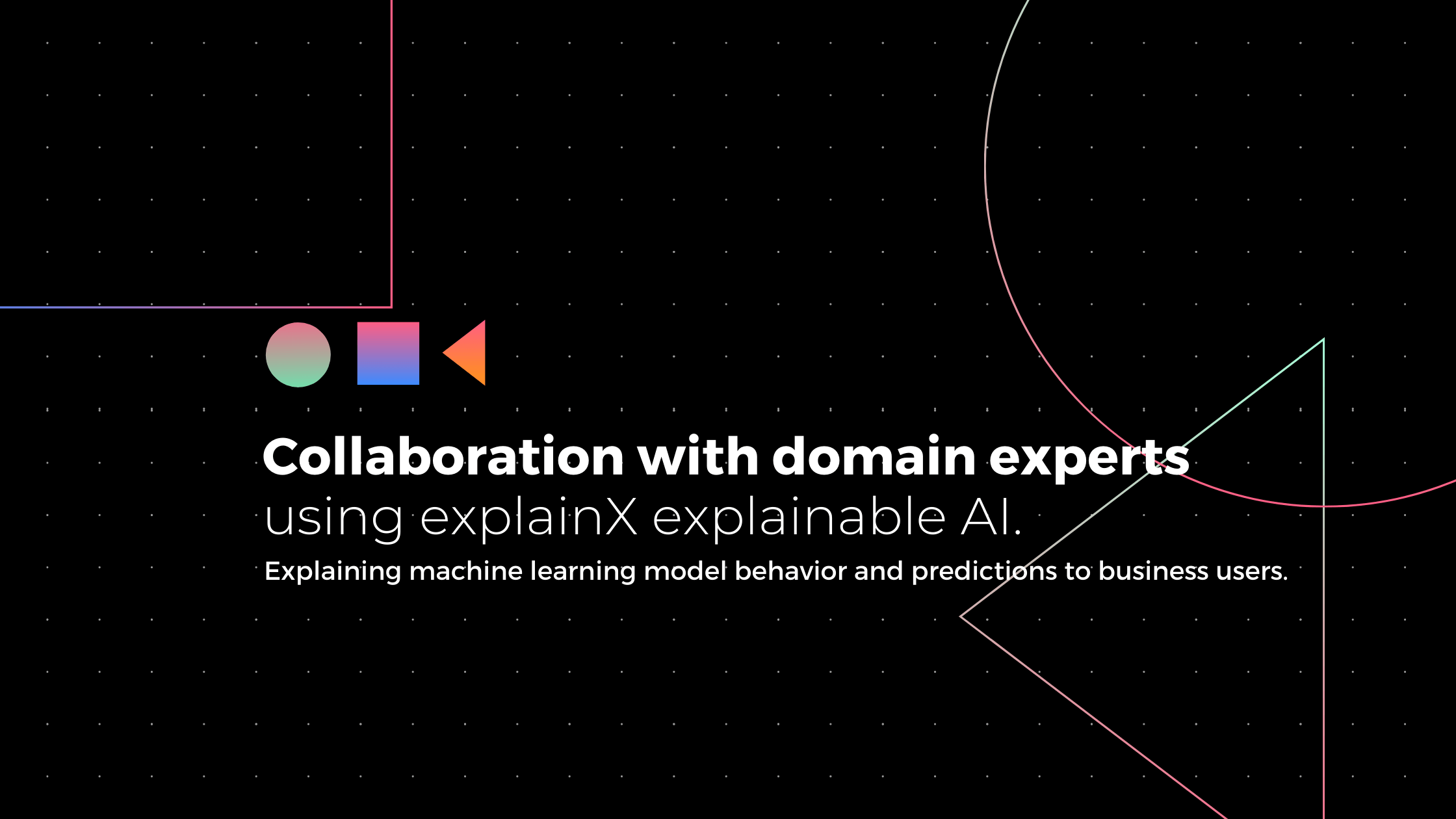Use Case & Tutorial

# How to convince domain experts to trust your blackbox model?

Post by
R&D TeamAfter building the Machine Learning model, there are two main questions that data scientists have to answer.

1. What is the overall logic of the model in making decisions?
2. Is the logic consistent with the domain knowledge, so that we can deploy the model with confidence?

To answer these questions, Data scientists not only have to understand the behavior of the model as a whole but also need to present the logic behind the model to domain experts to see how the model compares to the expert's knowledge. This comparison is really important as we want our model to focus on the right variables to make a prediction.

Explainx allows domain experts and model builders to collaborate(work as a team) to understand the logic of the model and decide if the logic of the model is reasonable.

There are three different levels of model explanations that domain experts really like.

1. Overall or global-level explanation.
2. Region or subset-level explanations.
3. Local or row-level explanations.

Explain, by default, gives global level explanations. This can be fund by aggregation of explanations of each row.

For the global level explanations, there are three important plots.

The first plot is feature importance that domain experts really like and I am pretty sure that data scientists are already aware of this.

This shows which features are given more attention by the model. This is really important because you don’t want the model to give low importance to a variable that domain experts think is important.

The next plot is called feature impact, which is just an extension of the feature importance plot. This gives us additional insight into whether the average importance of the variable is positive or negative. Negative importance here means most values of the variable will decrease the likelihood(probability) of getting a certain prediction.

It is important to note that some values of a variable might increase and some values might decrease the likelihood of getting a certain prediction. That is why Global level explanations also include Global level feature explanations in the form of the PDP plot.

Domain experts can look at this graph and validate whether this feature level trend makes sense to them or not.

Now let’s move on to region level explanations, which is based on the fact that the model might behave differently on different subsets of data.

Using the SQL box, we can define the region for which we need a model explanation, and let’s say our subset(region) is ExternalRiskestimate> 85.

All three global level plots will now change to explain the ML model on the defined region.

Now, the feature importance graph will show what variables/features are important when Risk estimate> 85, the feature impact graph will show if features are negatively or positively important, and PDP will show how the likelihood of getting a prediction changes with the change in the value of the feature.

Lastly, every domain expert wants to know how the ML model will perform in different scenarios. Local or row-level explanations that explainx provides will come handy here as it gives domain experts the power to define different scenarios and understand how the model is coming up with the prediction.

Let’s say domain experts want to explain the first data point in the data. Two main methods can be used for this purpose are.

1. Local SHAP/ LIME.
2. ProtoDash

Let’s say the model is giving the predictions “0”, and the probability of getting “0” is 0.45. Local SHAP will explain how the model came up with a probability of 0.1.

SHAP shows that the variable ExternalRiskEstimate with the value “61” has the impact value  -0.09, which means this variable will decrease the likelihood of getting “0” by -0.09

And on the other hand, variable NewFranctionRevolvingBurden with the value “0”  has an impact value 0.04, which means this variable will increase the probability of getting 0 by 0.04.

Adding all impact values generated by SHAP will give us the probability of 0.1 for getting “0”.

What will happen to the prediction if we change the value of one variable? What-if form that allows you to play will variables to see how the prediction changes.

The other method for row-level explanations is ProtoDash. ProtoDash answers a really important question. How is the model performing on data points similar to the data point that we want to explain? If the model is predicting something different on similar data points, then there is something wrong with the model.

ProtoDash can also help find similar data points with the same predictions. These similar points justify domain experts that the model predicted “default” because the model also predicted “default” on these similar data points.

In short, to make sure that the model doesn’t fail in production, it is important to make sure that the ML model is aligned with the domain experts’ understanding of how the world works. ML model’s success goes up tremendously if domain experts are happy with global-level, regional-level, and local-level explanations.

So if you're ready to convince domain experts to trust your model and you want to do it quickly and confidently, sign up for a free explainX account and explain your first model.